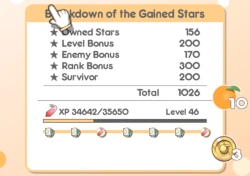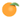# Co-op Reward ModifierCo-op mode uses a special reward modifier calculation to determine how much experience the player gets toward their role and how many oranges they will receive at the end of the match. This topic is touched on the co-op bosses and mixers page, but not thoroughly covered in-depth. This page explains the exact equation in finer detail.

## Difficulty Modifier

The first reward modifier is which boss difficulty the player selected. Each difficulty provides a percentage that effects the number ofOranges and amount of role experience. The base values within the game's code for a co-op game with no mixers is as follows below. (This is not to be confused with the actual value the player receives. These are the base values the equation uses, not the amount received.):

Boss Difficulty Oranges Base Role EXP Base
Casual 4 75
Normal 6 100
Extreme 7 125
Hyper 9 150

There is much more than meets the eyes to the table above, however. As previously mentioned, a calculation is at play. This calculation is the the base value of experience and oranges the player would receive from a normal match with no mixers multiplied to the difficulty modifier and then rounded down. The base number for oranges and role experience is 6 and 100 and respectively. Here is a table illustrating an explanation of how the calculation were done to get the values in the above table:

Boss Difficulty Difficulty Modifier Orange Calculation Role EXP Calculation
Casual -25% 6-(6*25%)= 4.5 100-(100*25%)= 75
Normal - 6 100
Extreme +25% 6+(6*25%) =7.5 100+(100*25%)= 125
Hyper +50% 6+(6*50%) =9 100+(100*50%)= 150

## Mixer Modifier

The second reward modifier is the mixers that are in play during the match. If the player does not have mixers active, this modifier is an automatic 0%. All the mixer percents are added together to equal the mixer modifier. Please refer to this page for additional information about the type of all mixers.. Below is a table of the mixer difficulties percentages:

Type Reward Modifier
Positive +0%
Neutral +10%
Negative +25%

Based on the table, the maximum mixer difficulty modifier the player can have is 75% if they receive three negative mixers. Please see the mixer page to see which mixers are positive, negative, or neutral.

## Loss Modifier

The third reward modifier is the loss modifier, which is based on if the player wins or loses. If the players successfully defeat the co-op boss and win the game, this modifier is an automatic 120%. If the players lose, the formula applies. The formula for this process uses the base value 3.333% which represents 1 of 30 chapters. This value is multiplied to the number of chapters the players survived. This only up to a maximum of 30 chapters, however, and any chapter after 30 will be treated as 30 in the equation. The result is a percent which acts as the loss modifier, and it is rounded down. Below is a table with examples of this calculation:

 Lost on Chapter Calculation Loss Modifier 5 3.333*5= 16.665% 16% 11 3.333*11= 36.666% 36% 26 3.333*26= 86.666% 86% 30 3.333*30= 100% 100% 36 3.333*30= 100% 100% (Game won) - 120%

## Full Oranges Calculation

To calculate the total number of oranges that all players will receive, all the modifiers are added together and multiplied with the base value to form the following equation:

```Total Oranges = (6 * loss modifier) * (100% + difficulty modifier + mixer modifier)
```

Below are some examples of the equation in action and the exact number of oranges the players will receive after winning or losing:

Difficulty Selected Types of Mixers Win/Loss Calculation Oranges
Casual - Win (6 * 120%) * (100% + (-25%) + 0%) = 5.4 5
Normal - Win (6 * 120%) * (100% + 0%) = 7.2 7
Extreme - Win (6 * 120%) * (100% + 25% + 0%) = 9 9
Hyper - Win (6 * 120%) * (100% + 50% + 0%) = 10.8 10
Casual 3 negatives Win (6 * 120%) * (100% + (-25%) + [25%*3]) = 10.8 10
Normal 1 negative/1 neutral Win (6 * 120%) * (100% + [25% + 10%]) = 9.72 9
Normal 1 neutral Loss on Chapter 11 (6 * 36%) * (100% + 10%) = 2.376 2
Extreme 2 negative/1 neutral Win (6 * 120%) * (100% + 25% + [25%*2 + 10%]) = 13.32 13
Extreme 3 neutral Win (6 * 120%) * (100% + 25% + [10%*3]) = 11.16 11
Extreme 1 negative/2 neutral Loss on Chapter 31 (6 * 100%) * (100% + 25% + [25% + 10%*2]) = 10.2 10
Hyper 1 negative Win (6 * 120%) * (100% + 50% + 25%) = 12.6 12
Hyper 2 positive Loss on Chapter 21 (6 * 69%) * (100% + 50% + 0%) = 6.21 6
Hyper 3 negative Win (6 * 120%) * (100% + 50% + [25%*3]) = 16.2 16

## Full EXP Calculation

To calculate the total amount of co-op role experience that all players will receive, all the modifiers are added together and multiplied with the base value to form the following equation:

```Total Experience = (100 * loss modifier) * (100% + difficulty modifier + mixer modifier)
```

Below are some examples of the equation in action and the exact amount of experience the players will receive after winning or losing:

Difficulty Selected Types of Mixers Win/Loss Calculation Role EXP
Casual - Win (100 * 120%) * (100% + (-25%) + 0%) = 90 90
Normal - Win (100 * 120%) * (100% + 0%) = 120 120
Extreme - Win (100 * 120%) * (100% + 25% + 0%) = 150 150
Hyper - Win (100 * 120%) * (100% + 50% + 0%) = 180 180
Casual 3 negatives Win (100 * 120%) * (100% + (-25%) + [25%*3]) = 180 180
Normal 1 negative/1 neutral Win (100 * 120%) * (100% + [25% + 10%]) = 162 162
Normal 1 neutral Loss on Chapter 11 (100 * 36%) * (100% + 10%) = 39.6 39
Extreme 2 negative/1 neutral Win (100 * 120%) * (100% + 25% + [25%*2 + 10%]) = 222 222
Extreme 3 neutral Win (100 * 120%) * (100% + 25% + [10%*3]) = 186 186
Extreme 1 negative/2 neutral Loss on Chapter 31 (100 * 100%) * (100% + 25% + [25% + 10%*2]) = 170 170
Hyper 1 negative Win (100 * 120%) * (100% + 50% + 25%) = 210 210
Hyper 2 positive Loss on Chapter 21 (100 * 69%) * (100% + 50% + 0%) = 103.5 103
Hyper 3 negative Win (100 * 120%) * (100% + 50% + [25%*3]) = 270 270

## Calculator

Match outcome Win Loss at chapter or later Casual Normal Extreme Hyper Enable mixers None/Positive Neutral Negative None/Positive Neutral Negative None/Positive Neutral Negative 7 120